Next: LASERQED Up: Commands Previous: BEAM

## LASER

Defines a laser. There can be upto 5 lasers but this can easily be increased. One LASER command defines one laser. Note that lasers, if there are more than one, act incoherently. Their interference effects cannot be included in the present version.

The longitudinal (time) pulse shape can be Gaussian or trapezoidal but the transverse is Gaussian only. LASER [RIGHTLEFT,] WAVELENGTH=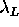, POWERDENSITY=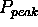, [TXYS=(t,x,y,s),] E3=(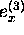,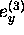,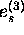), E1=(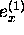,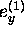,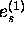), RAYLEIGH=(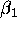,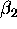), [GCUT=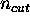,] SIGT=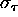TTOT=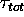, [GCUTT=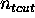,] [EDGE=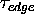,] [STOKES=(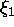,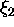,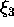),] ;

RIGHTLEFT
Right-going or left-going. If RIGHT(LEFT) is specified, the laser acts only onto the left(right)-going particles (to save computing time). If omitted, acts on both.Laser wavelength (m).Peak power density (Watt/m).
t,x,y,s
Laser focal point and the time when the laser pulse comes there.
(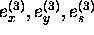)
Unit vector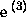along the direction of laser propagation.
(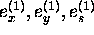)
Unit vector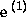perpendicular to.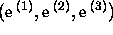with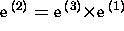forms a right-handed orthonormal frame.andneed not be normalized exactly and need not be perpendicular to each other exactly.,Rayleigh length  in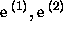direction. (meter)Cut off of transverse tail tail in units of sigmas. Default=2.5.R.m.s. pulse length (times velocity of light) in power, not in field amplitude, assuming Gaussian structure. (meter)Cut off of longitudinal tail in units of sigmas for Gaussian time structure. Default=2.5.Total pulse length for trapezoidal longitudinal structure (meter). Either one of SIGT or TTOT must be specified.Longitudinal edge length (meter) for trapezoidal time structure. The flat-top length is then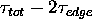. Default=0 (i.e., rectangular shape).,,Stokes parameter   defined in theframe. Default=(0,0,0).

See Sec.5.7.1 for more detail.

Toshiaki Tauchi
Thu Dec 3 17:27:26 JST 1998